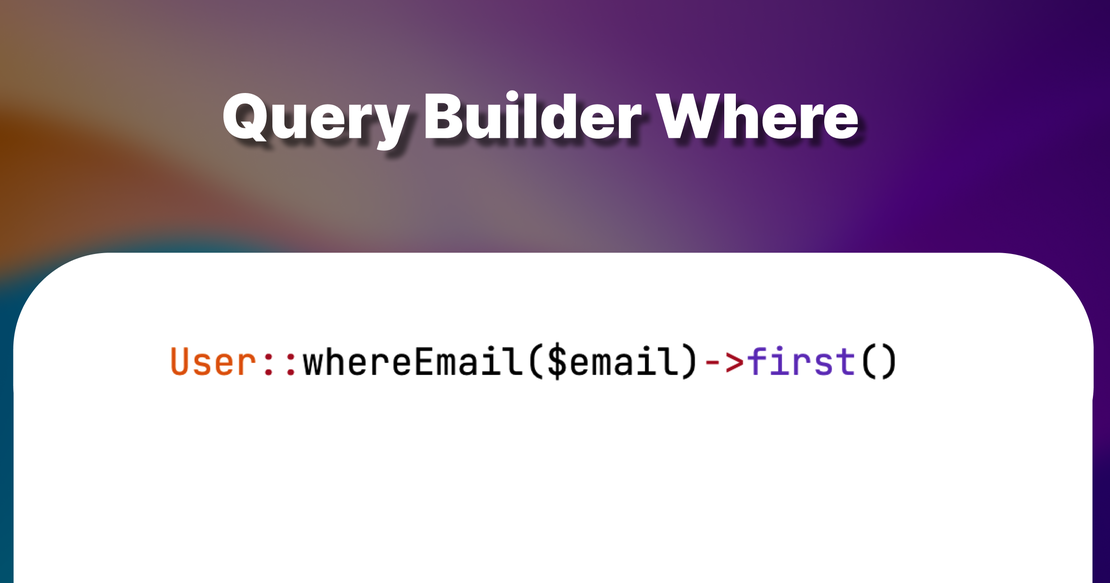# Laravel Where## Introduction

The simples way to use `where` is just to statically call it on your model as `Model::where('name', 'Ahmed')->first()`

Laravel Query builder is very powerful. It offers a rich set of methods that makes it easy for you to write SQL queries in a very easy way.

One of those methods is the Where method and it has many ways to simplify complex queries

## The Query Builder

First of all, you need to understand that where and all its siblings lives in the Builder class. and when you call a static method on the model directory. Most of the times, it will be forwarded to the Eloquent query builder

``````1public static function __callStatic(\$method, \$parameters)
2{
3    return (new static)->\$method(...\$parameters);
4}
``````
`````` 1public function __call(\$method, \$parameters)
2{
3    if (in_array(\$method, ['increment', 'decrement', 'incrementQuietly', 'decrementQuietly'])) {
4        return \$this->\$method(...\$parameters);
5    }
6
7    if (\$resolver = \$this->relationResolver(static::class, \$method)) {
8        return \$resolver(\$this);
9    }
10
11    if (Str::startsWith(\$method, 'through') &&
12        method_exists(\$this, \$relationMethod = Str::of(\$method)->after('through')->lcfirst()->toString())) {
13        return \$this->through(\$relationMethod);
14    }
15
16    return \$this->forwardCallTo(\$this->newQuery(), \$method, \$parameters);
17}
``````

The forwardCallTo, forwards the method call to the `\$this->newQuery()` which returns an instance of Builder class

``````1/**
2 * Get a new query builder for the model's table.
3 *
4 * @return \Illuminate\Database\Eloquent\Builder
5 */
6public function newQuery()
7{
8    return \$this->registerGlobalScopes(\$this->newQueryWithoutScopes());
9}
``````

So by looking into the `where` method in the Builder class. it’s about 100 lines . That’s because it can handle many cases. So let’s take them one by one

### Simple where condition

The first easy simple way to write a condition in laravel is to just pass the key and value to the where.

``````1User::where('email', 'root@admin.com')->first()
``````

This will translate to

``````1SELECT * FROM users WHERE `email` = 'root@admin.com' LIMIT 1
``````

### Multiple where

#### Chain wheres

You can also chain several wheres

``````1User::where('email', 'root@admin.com')
2	->where('is_active', true)->first()
``````

This will translate to

``````1SELECT * FROM users WHERE `email` = 'root@admin.com' AND `is_active` = true LIMIT 1
``````

#### Array of Wheres

Another way to do so is to use an array of where

``````1User::where([
3	'is_active' => true
4])->first()
``````

This will translate to

``````1SELECT * FROM users WHERE `email` = 'root@admin.com' AND `is_active` = true LIMIT 1
``````

### Using OrWhere

The OrWhere method is used to add an OR constraint to your queries. It functions similarly to the where method, but adds the condition as an “OR” clause rather than an “AND” clause.

``````1User::where('email', 'root@admin.com')
``````

But how we do the `is_active` when we use OR?

### Grouped Conditions

We can use grouped conditions by passing a closure to the where method

``````1User::where(function(\$q) {
4})
5->where('is_active', true)
6->first()
``````

And this will translate to

``````1SELECT
2*
3FROM `users`
5AND `is_active` = 1
6
7LIMIT 1
``````

And you can have as many nested groupings as you want

### When and Where

You can also apply specific wheres only when a condition is true

``````1\$onlyActive = true;
2
4->when(\$onlyActive, function(\$q) {
5	\$q->where('is_active', true);
6})->first()
``````

The where is_active = true will be only applied if the `\$onlyActive` is true

### Shortcuts

There are also some shortcuts that can be applied to simplify how you write eloquent

#### Where{Column}

``````1User::whereEmail('root@admin.com')->first()
``````

Translates to

``````1SELECT * FROM `users` WHERE `email` = 'root@admin.com' LIMIT 1
``````

#### Where{Column}And{Column}

``````1User::whereEmailAndStatus('root@admin.com','active')->first()
``````

Translates to

``````1SELECT * FROM `users` WHERE `email` = 'root@admin.com' AND `status` = 'active' LIMIT 1
``````

#### Where{Column}Or{Column}

``````1User::whereEmailOrUserName('root@admin.com','admin')->first()
``````

Translates to

``````1SELECT * FROM `users` WHERE `email` = 'root@admin.com' OR `user_name` = 'admin' LIMIT 1
``````

### WhereNull

You can also look for records where the column value is null

``````1User::whereNull('confirmed_at')->get()
``````
``````1SELECT * FROM `users` WHERE `confirmed_at` IS NULL
``````

### WhereNot

There is also `WhereNot` to apply “NOT EQUAL TO” condition in your queries.

``````1User::whereNot('status','active')->all()
``````

Which translates to

``````1SELECT * FROM `users` WHERE NOT `status` = 'active'
``````

### WhereNotNull

A combination of the previous two

``````1User::whereNotNull('confirmed_at')->all()
``````

Which translates to

``````1SELECT * FROM `users` WHERE `confiremd_at` IS NOT NULL
``````

### Conclusion

We checked together what are the possible ways to use the where method. Let me know if you have any tips or tricks or maybe missed cases I did not cover in the comments.